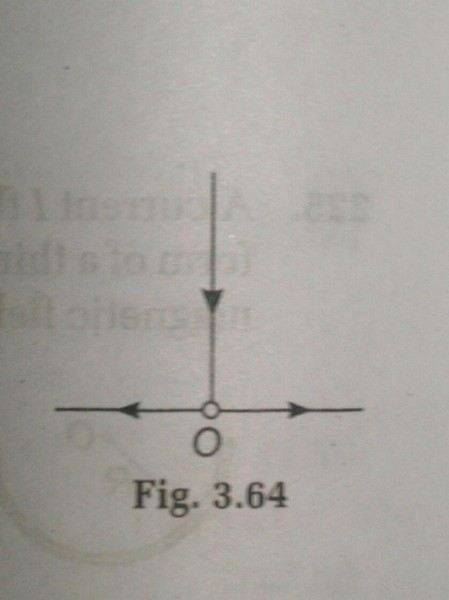# Magnetic field due to wire and conducting plane

bsdnoob
< Mentor Note -- thread moved to HH from the technical physics forums, so no HH Template is shown >

This problem is bugging me since a week so I decided to post this here

Suppose we have a wire with some current 'I' and which at a point 'o' spreads radially in all direction along conducting plane perpendicular to wire so what will be magnetic field at above and below plane?

Here's the diagramMy approach to this problem was to find magnetic field due to individual current carrying elements . For wire I used Ampere's law to find it as B= (mu) . I /4πx (for half infinite wire)but I am confused about it from conducting plane ? Answer is given to be 0 for region below plane , how is this possible? And for above plane is given (mu)I/2πx but how? can someone help?
This problem is given in irodov's general physics book. (3.232)

Last edited by a moderator:

Bystander
Homework Helper
Gold Member
(for half infinite wire)
Why half?

TSny
Homework Helper
Gold Member
Using Ampere's law sounds like a good approach. Can you explain in detail how you set up Ampere's law to get your answer?